How to do Fractions the Easy Way

Understanding Fractions (the easy way!)
 Fractions

Fractions can be thought of as another way of seeing division. If you have one whole pizza and two people want to share it equally, they will divide it into two halves: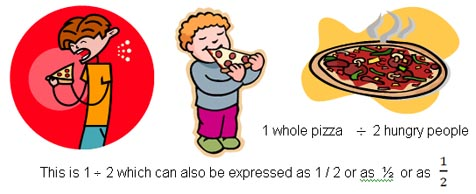The bottom number of a fraction is the denominator. It tells how many parts a whole has been divided into. In this case our pizza is divided into two parts.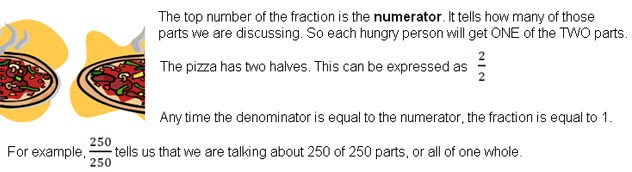Proper Fractions, Improper Fractions, and Mixed Numbers

A proper fraction is one in which the numerator is smaller than the denominator.
An improper fraction is one in which the numerator is larger than the denominator. If the numerator is larger than the denominator, the fraction equals a number that is greater than one.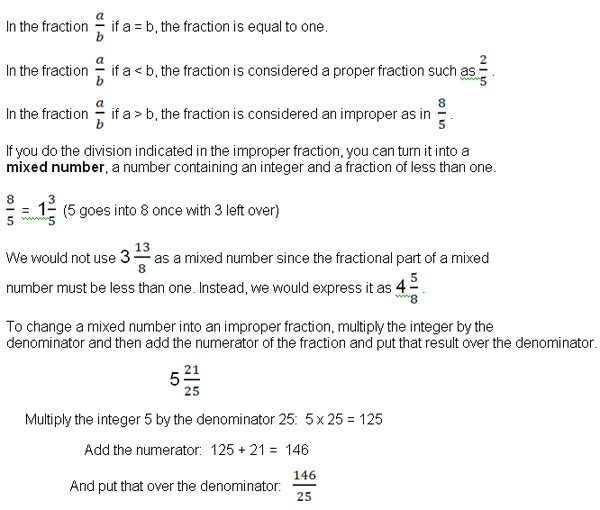Lowest Terms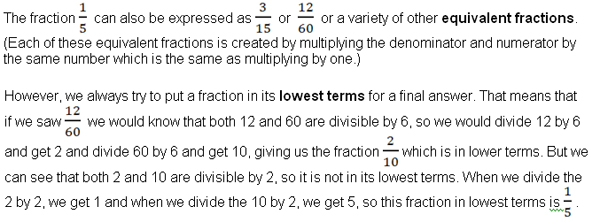Finding the GCF (Greatest Common Factor)

The greatest common factor is the largest factor that divides two numbers. We find this by figuring out what prime numbers are multiplied together to make up each number. A prime number is a number that can only be divided by itself or by one. Examples of prime numbers are 1, 2, 3, 5, 7, 11, 13, 17, 19, 23, 29, etc. We want to focus on those that are less than ten in most cases.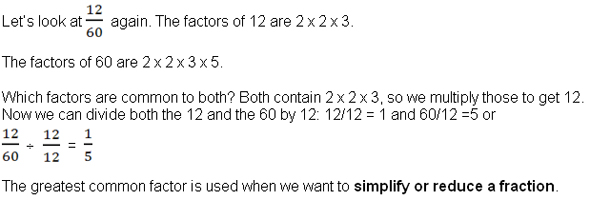Interested in learning more? Why not take an online Basic Math course?
 Finding the LCD (Lowest Common Denominator)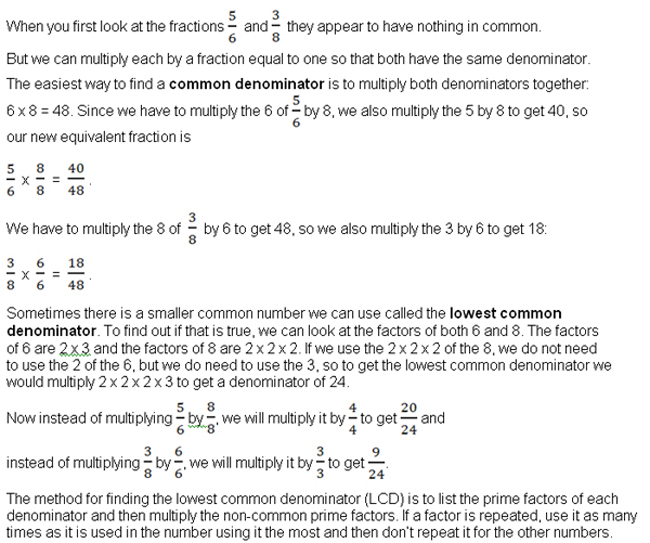To add or subtract two fractions, the two fractions must have the same denominator. Therefore, you will have to find the lowest common denominator and change each fraction to an equivalent fraction. Then you add or subtract the numerators as indicated and put the resultant number over the denominator and reduce the fraction to lowest terms.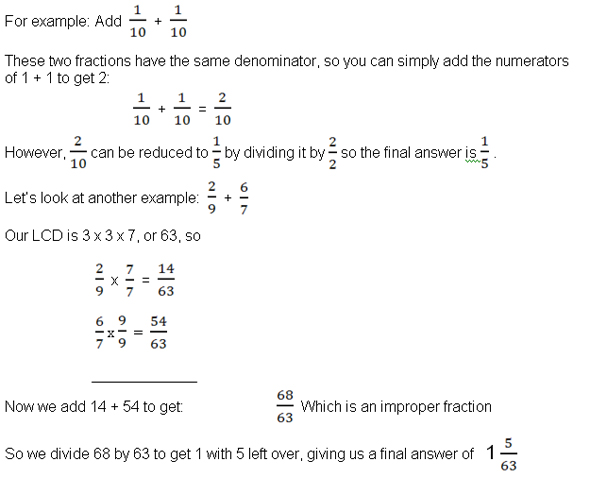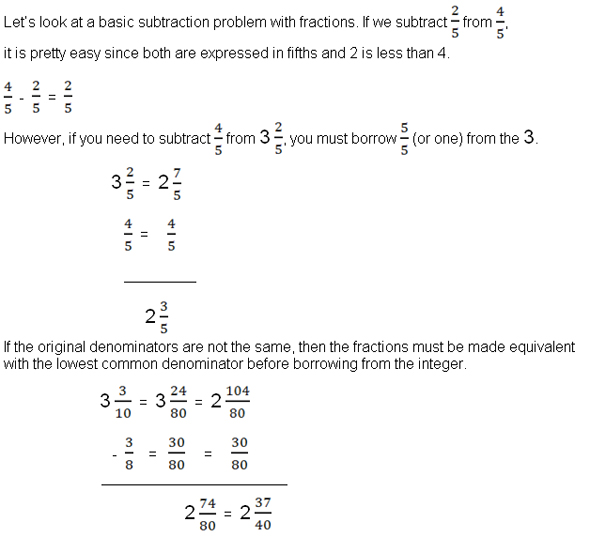Multiplying and Dividing Fractions

Multiplying fractions is fairly simple. You may want to put each fraction in its lowest terms to start. Then multiply the numerators to get a numerator and multiply the denominators to get a new denominator. And reduce or simplify the fraction to put it in its lowest terms. If you are multiplying a fraction by an integer, put the integer over one to make a fraction of it.

Examples:

Five times 4/5: Put the five over one, then multiply 5 x 4 to get 20 and 1 x 5 to get 5. Twenty can be divided evenly by 5 to give us an answer of 4.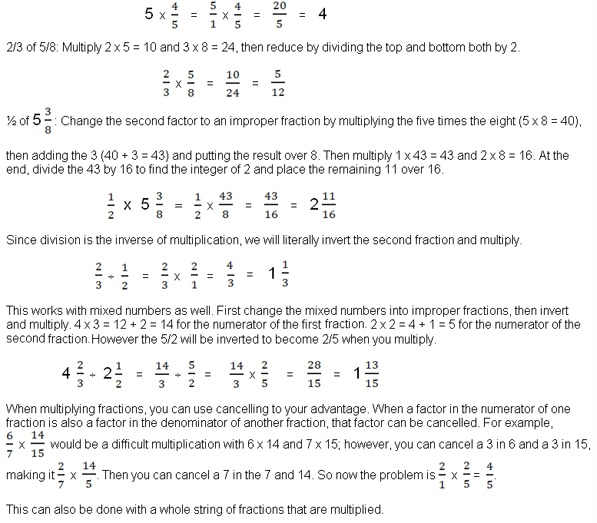Reciprocals of Fractions

The product of a number and its reciprocal equals 1.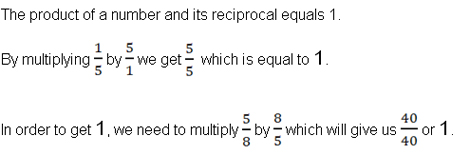Signs and Fractions

If either the denominator or numerator is negative, the fraction is considered a negative fraction. If both the denominator and the numerator are negative, the fraction is considered to be a positive fraction.

Adding two fractions of the same sign (either positive or negative) gives an answer with the same sign. You may need to give the fractions common denominators first.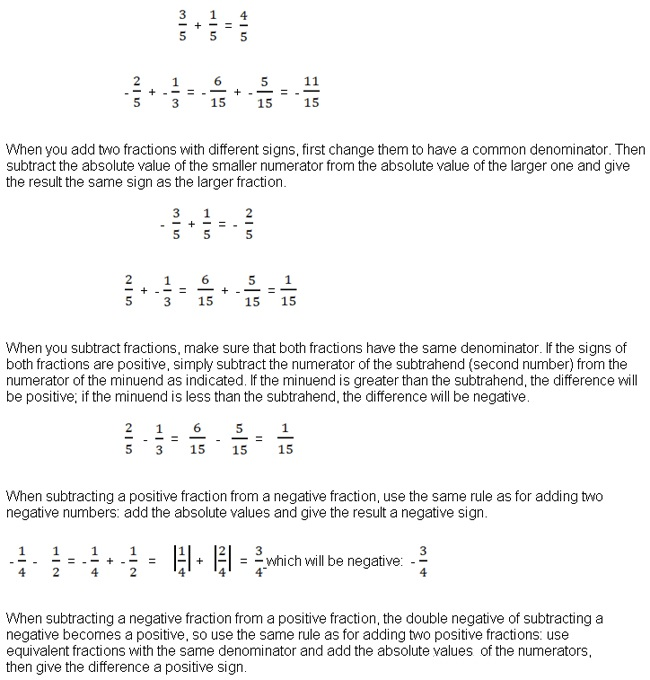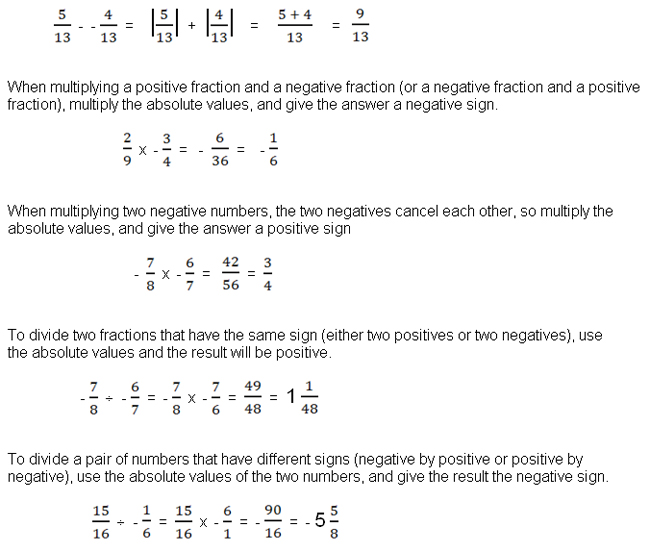Are you looking for more help with fractions?  Check out this article on how to add, subtract, multiply and divide fractions. Also consider taking an online course in basic math or pre-algebra to brush up your math skills.